## Delta Function Potentials p.10

### Projects for you!

The below is a list of project you should be able to complete. For some of the projects Mathematica may be of use. Here's a file containing the Mathematica I used to make these pages.

1. Another famous formula involving the Dirac delta function is:Describe how the units work out in this equation. Give a qualitative discussion as to why this integral is zero unless k=k' (in which case it's infinite).

2. A narrow, deep square well should approximate a delta function. Show how our square well solutions go over to our delta function solution in the limit of a deep, narrow well.

3. For scattering from a "one-nuclei" delta function, we found the reflection amplitude:

R = -1/(1+ik)

For a square well potential we found the reflection amplitude to be: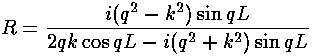Now a narrow, deep square well should approximate a delta function. Show that in this limit the above two formulae agree.

4. Consider a variational approximation to solving the "one-nuclei" bound state problem. Try a gaussian trial wavefunction:= exp(-ax2)

with the adjustable parameter a. Try another function of your own choosing.

5. Use the graphs

a = s(tanh(s)+1)

a = s(coth(s)+1)

on page 4 to estimate the bound state energies for a diatomic molecule a=1,2,3,4. Find those energies directly with a root finder. How do the two methods compare?

6. We worked out the quantum mechanical motion of the N=8 molecule. The two state (say a=4) molecule has quite interesting "motion"...work it out. What is the period? Can you relate the period to quantum mechanical variables?

7. Write down the order of the lowest four H2+ energy levels for a=10. What are the next three levels?
1. The fourth state on your list should be:u. Describe why, under inversion between the nuclei, the label "u" is deserved. Describe this state!
2. Earlier we have worked out the energy levels of a 2D infinite circular square well. It is trivial to extend this model to a cylinder. Find the aspect ratio (length/radius) of a cylinder which produces the same ordering of states.
3. Consider the aufbau of N2, O2, and F2. Show how the electrons are distributed for N2. I claim O2 has unpaired electrons. What does that say about the state beyond that used for N2? Show how the electrons are distributed for F2.

8. Approximate the ground state energy of a H2+ molecule by the variational method. Use as your trial function:

exp(-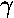r1) + exp(-r2)

where r1 is the distance from one nuclei and r2 is the distance from the other. Plot the results as a function of a and compare to the exact result displayed on page 4.

9. Approximate the energy of the firstu orbital energy by the variational method. Use the above wavefunction multiplied by:

exp(i)

What is the justification for using the variational method to find an excited state energy? Plot the results as a function of a and compare to the exact result displayed on page 4.

10. Consider the simple 1D molecule with repulsion:Find the "spring constant" that defines the motion near equilibrium. Assume the nuclei weigh 2000 times the elections. Find the frequency of oscillation in our dimensionless units. Don't forget reduced mass, and be careful working out the units!

11. Consider the real H2 molecule: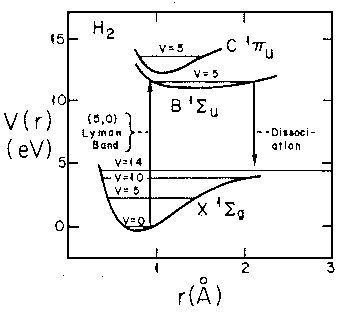Find the "spring constant" that defines the motion near equilibrium. Assume the nuclei are protons. Find the frequency of oscillation in Hz. Don't forget reduced mass, and be careful working out the units!

12. Consider the theoretical H2+ molecule: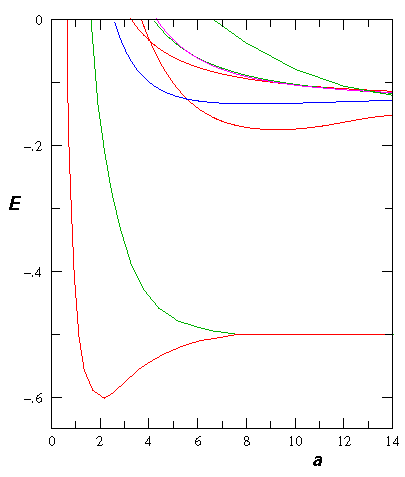Find the "spring constant" that defines the motion near equilibrium. Assume the nuclei are protons. Find the frequency of oscillation in Hz. Don't forget reduced mass, and be careful working out the units!

13. on page 5 I claim the N=3 molecule looses levels at a=3/2 and 1/2. Show this.

14. We separated the bands from the gaps on the basis of the magnitude of the eigenvalues: in the bands, where the wavefunction oscillates, ||=1 where as in the gaps, where we have decay or growth, we have one eigenvalue ||>1. The ability to make this separation was bases on the determinant of [M] being 1. For general potentials separated by free regions, show:

| det([M]) | = 1

15. Find [M] in the case of a nuclear potential given by a finite depth square well. Find the band structure for some particular well.

16. Consider a lattice where we double the cell size by moving every other nucleus to the left by some distance b:Find the band structure.

17. In the band structure pages, I stress the usefulness of studying a empty (free) lattice. Find the band structure of an empty 1D crystal, and compare to that we found with delta function nuclei.

18. Find the band structure of a lattice of repulsive (+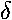) potentials.

19. Consider a particle in an infinite square well with a repulsive delta function at its center. Find the exact eigenenergies. Find the approximate eigenenergies by treating the delta function as a perturbation. For the ground state, calculate and numerically sum the second order perturbation contribution. Plot the results as a function of the strength of the delta function.

20. Consider the N=3 molecule with a=2. Using the below plot (or direct calculation) find the eigenenergies of the three levels. Find a finite square well whose energy level ratios approximate those found in the delta function case. Discuss the difficulty of doing the same thing for a=4.21. Investigate the spacing of the finite molecule energy levels within the energy band. Consider, for example, N=16, a=4 molecule. Find all its eigenenergies and plot the results on the band. Find the q that correspond to each energy level.

22. Consider a case like a=½ where the band spans E=0. Combine the E<0 band with the E>0 band into one plot.

23. Consider two adjacent bands accessible to scattering. One has negative group velocity and the other has positive group velocity. Using a relatively large crystal for example, N=32, create a superposition solution in which a lump of probability approaches the crystal with (a) energy in the negative group velocity band and (b) energy in the positive group velocity band. Describe the quantum motion in each case.